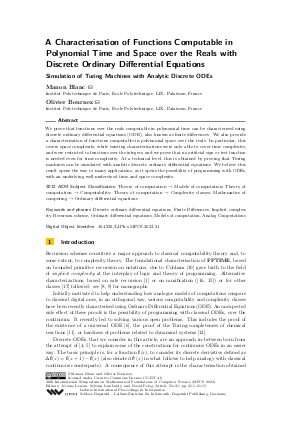Document# A Characterisation of Functions Computable in Polynomial Time and Space over the Reals with Discrete Ordinary Differential Equations: Simulation of Turing Machines with Analytic Discrete ODEs

### Authors Manon Blanc, Olivier Bournez## File

LIPIcs.MFCS.2023.21.pdf
• Filesize: 0.88 MB
• 15 pages

## Cite As

Manon Blanc and Olivier Bournez. A Characterisation of Functions Computable in Polynomial Time and Space over the Reals with Discrete Ordinary Differential Equations: Simulation of Turing Machines with Analytic Discrete ODEs. In 48th International Symposium on Mathematical Foundations of Computer Science (MFCS 2023). Leibniz International Proceedings in Informatics (LIPIcs), Volume 272, pp. 21:1-21:15, Schloss Dagstuhl - Leibniz-Zentrum für Informatik (2023)
https://doi.org/10.4230/LIPIcs.MFCS.2023.21

## Abstract

We prove that functions over the reals computable in polynomial time can be characterised using discrete ordinary differential equations (ODE), also known as finite differences. We also provide a characterisation of functions computable in polynomial space over the reals. In particular, this covers space complexity, while existing characterisations were only able to cover time complexity, and were restricted to functions over the integers, and we prove that no artificial sign or test function is needed even for time complexity. At a technical level, this is obtained by proving that Turing machines can be simulated with analytic discrete ordinary differential equations. We believe this result opens the way to many applications, as it opens the possibility of programming with ODEs, with an underlying well-understood time and space complexity.

## Subject Classification

##### ACM Subject Classification
• Theory of computation → Models of computation
• Theory of computation → Computability
• Theory of computation → Complexity classes
• Mathematics of computing → Ordinary differential equations
##### Keywords
• Discrete ordinary differential equations
• Finite Differences
• Implicit complexity
• Recursion scheme
• Ordinary differential equations
• Models of computation
• Analog Computations

## Metrics

• Access Statistics
• Total Accesses (updated on a weekly basis)
0

## References

1. Stephen Bellantoni and Stephen Cook. A new recursion-theoretic characterization of the poly-time functions. Computational Complexity, 2:97-110, 1992.2. Manon Blanc and Olivier Bournez. A characterization of polynomial time computable functions from the integers to the reals using discrete ordinary differential equations. Submitted. Journal version of [Manon Blanc and Olivier Bournez, 2022]. Preliminary version available on URL: https://arxiv.org/abs/2209.13599.
3. Manon Blanc and Olivier Bournez. A characterization of polynomial time computable functions from the integers to the reals using discrete ordinary differential equations. In Jérôme Durand-Lose and György Vaszil, editors, Machines, Computations, and Universality - 9th International Conference, MCU 2022, Debrecen, Hungary, August 31 - September 2, 2022, Proceedings, volume 13419 of Lecture Notes in Computer Science, pages 58-74. Springer, 2022. MCU'22 Best Student Paper Award. URL: https://doi.org/10.1007/978-3-031-13502-6_4.
4. Olivier Bournez and Arnaud Durand. Recursion schemes, discrete differential equations and characterization of polynomial time computation. In Peter Rossmanith, Pinar Heggernes, and Joost-Pieter Katoen, editors, 44th Int Symposium on Mathematical Foundations of Computer Science, MFCS, volume 138 of LIPIcs, pages 23:1-23:14. Schloss Dagstuhl - Leibniz-Zentrum für Informatik, 2019.5. Olivier Bournez and Arnaud Durand. A characterization of functions over the integers computable in polynomial time using discrete ordinary differential equations. Computational Complexity, 32(2):7, 2023.6. Olivier Bournez and Amaury Pouly. A universal ordinary differential equation. In International Colloquium on Automata Language Programming, ICALP'2017, 2017.7. Vasco Brattka, Peter Hertling, and Klaus Weihrauch. A tutorial on computable analysis. In New computational paradigms, pages 425-491. Springer, 2008.8. P. Clote. Computational models and function algebras. In Edward R. Griffor, editor, Handbook of Computability Theory, pages 589-681. North-Holland, Amsterdam, 1998.9. Peter Clote and Evangelos Kranakis. Boolean functions and computation models. Springer Science & Business Media, 2013.10. Alan Cobham. The intrinsic computational difficulty of functions. In Y. Bar-Hillel, editor, Proceedings of the International Conference on Logic, Methodology, and Philosophy of Science, pages 24-30. North-Holland, Amsterdam, 1962.11. Francois Fages, Guillaume Le Guludec, Olivier Bournez, and Amaury Pouly. Strong turing completeness of continuous chemical reaction networks and compilation of mixed analog-digital programs. In Computational Methods in Systems Biology-CMSB 2017, 2017. CMSB'2017 Best Paper Award.12. Daniel S. Graça and Ning Zhong. Handbook of Computability and Complexity in Analysis, chapter Computability of Differential Equations, pages 71-99. Springer, 2018.13. Ker-I Ko. Complexity Theory of Real Functions. Progress in Theoretical Computer Science. Birkhaüser, Boston, 1991.14. D. Leivant. Intrinsic theories and computational complexity. In LCC'94, number 960 in Lecture Notes in Computer Science, pages 177-194, 1995.15. Daniel Leivant. Predicative recurrence and computational complexity I: Word recurrence and poly-time. In Peter Clote and Jeffery Remmel, editors, Feasible Mathematics II, pages 320-343. Birkhäuser, 1994.16. Daniel Leivant and Jean-Yves Marion. Lambda calculus characterizations of Poly-Time. Fundamenta Informatica, 19(1,2):167,184, 1993.17. Daniel Leivant and Jean-Yves Marion. Ramified recurrence and computational complexity II: substitution and poly-space. In L. Pacholski and J. Tiuryn, editors, Computer Science Logic, 8th Workshop, CSL'94, volume 933 of Lecture Notes in Computer Science, pages 369-380, Kazimierz, Poland, 1995. Springer.18. David B Thompson. Subrecursiveness: Machine-independent notions of computability in restricted time and storage. Mathematical Systems Theory, 6(1-2):3-15, 1972.19. Klaus Weihrauch. Computable Analysis: an Introduction. Springer, 2000.X

Feedback for Dagstuhl Publishing